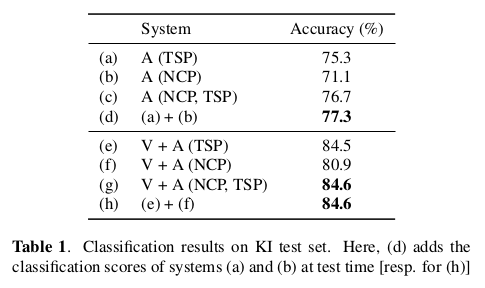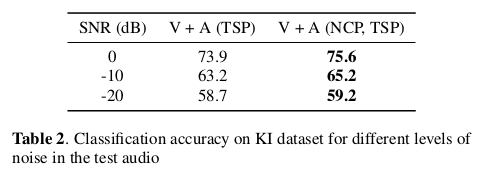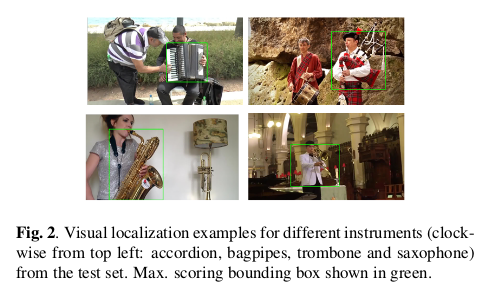# 论文引入

Weakly Supervised Representation Learning for Unsynchronized Audio-Visual Events一文将视频分解为图像区域和时间音频片段，在单独对视觉和音频子模块中处理每个视频，最后根据处理得到的视觉分类得分和听觉分类得分最终对场景进行分类。这个过程由下图所示：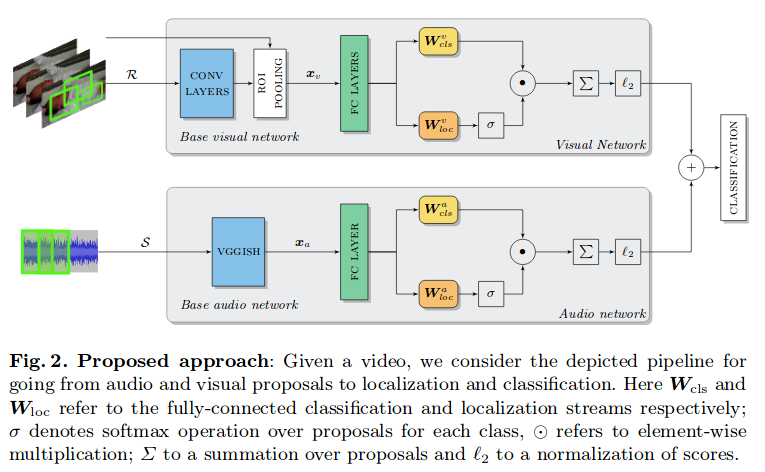• 通过视听场景分析对事件进行合理分类
• 通过音频处理上对音频的分离处理，增强了原有系统的鲁棒性
• 在视听场景弱标记数据集上定量和定性地验证系统的性能

# NMF介绍

The Sound of Pixels一文中，论文利用U-Net在真实掩码的训练下实现了声音的分离，这个方法的源头也是受到NMF的方法启发，这个我们在论文解读的博客中也有所提及。只依赖于弱标签进行声音的分离是Identify, locate and separate: Audio-visual object extraction in large video collections using weak supervision的一个创新点。

$Q \approx WH$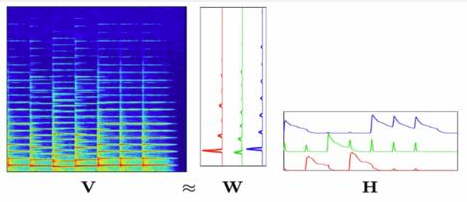# 模型框架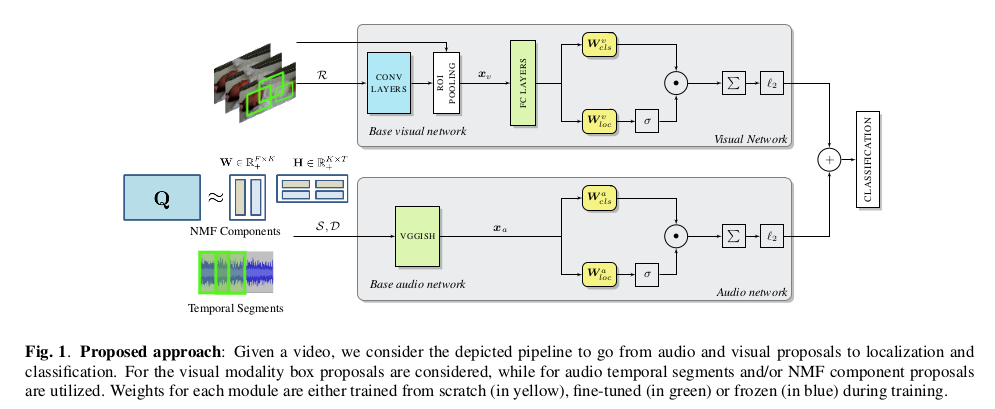$x_v$再通过两个全连接层，通过双流（twostream）架构，该模块的体系结构包括并行分类和定位流。前者通过使特征通过具有权重$W_{cls}$的线性全连接层来分类每个区域，得到矩阵$A \in \mathbb R^{\vert \mathcal p \vert \times C}$。另一方面，定位层通过权重$W_{loc}$通过另一个全连接层传递相同的输入。然后对定位流中的结果矩阵$B \in \mathbb R^{\vert \mathcal p \vert \times C}$进行softmax操作。

1.时间段（Temporal Segment Proposals (TSP)）：音频被简单地分解为相等长度的T个时间段$S=\lbrace s_1, s_2,…,s_T \rbrace$，log-Mel频谱图通过沿时间轴滑动固定长度窗口来对其进行分块而获得的。

2.NMF分量建议（NMF Component Proposals (NCP)）：使用NMF，我们分解由F频率区和N个短时傅里叶变换（STFT）帧组成的音频幅度谱图，具体的细节我们在前面的NMF中有过介绍。

$L(w) = \frac{1}{CN} \sum_{n=1}^N \sum_{c=1}^C max(0,1-y^{(n)} \phi_c(V^{(n)};w))$

# 实验